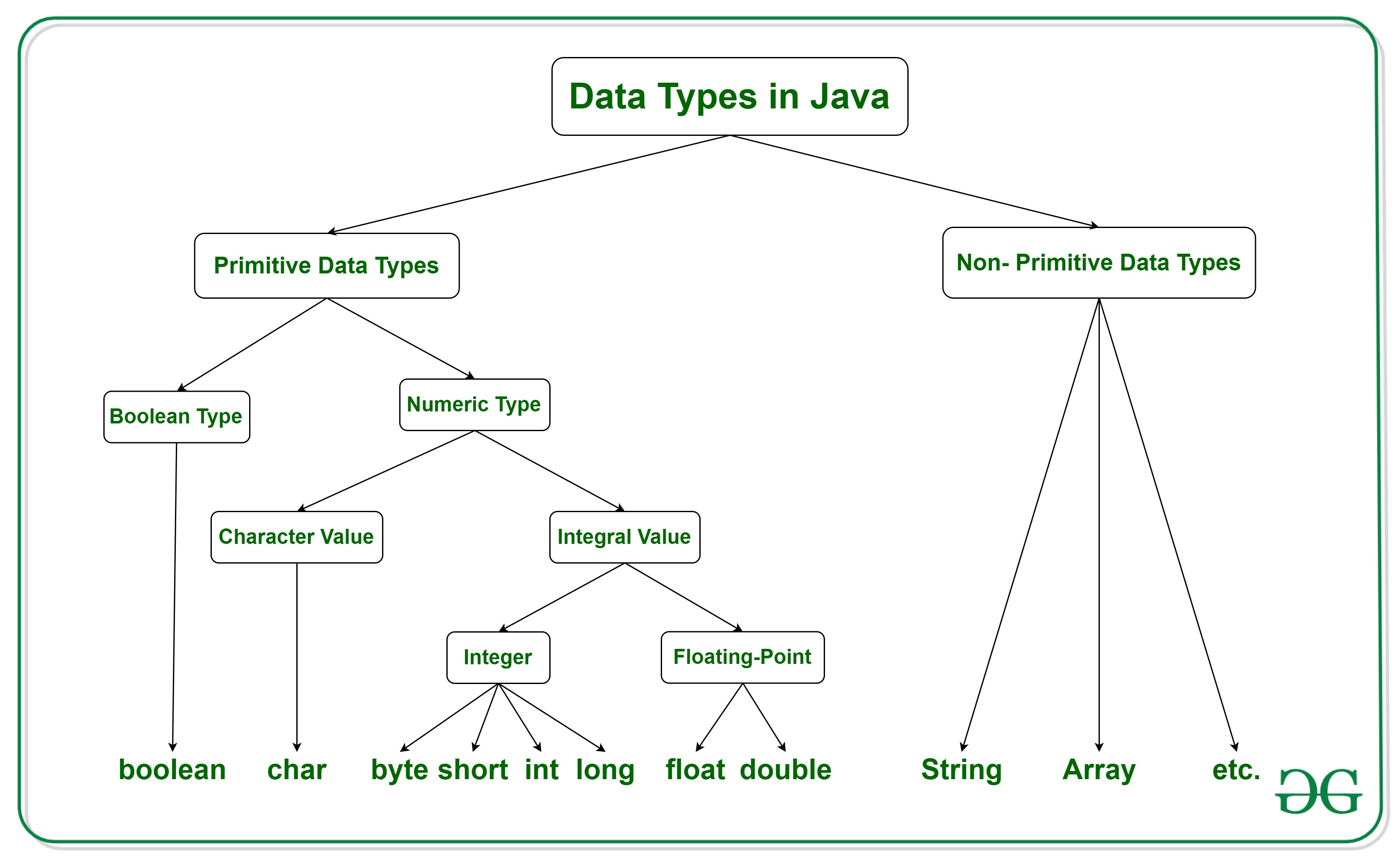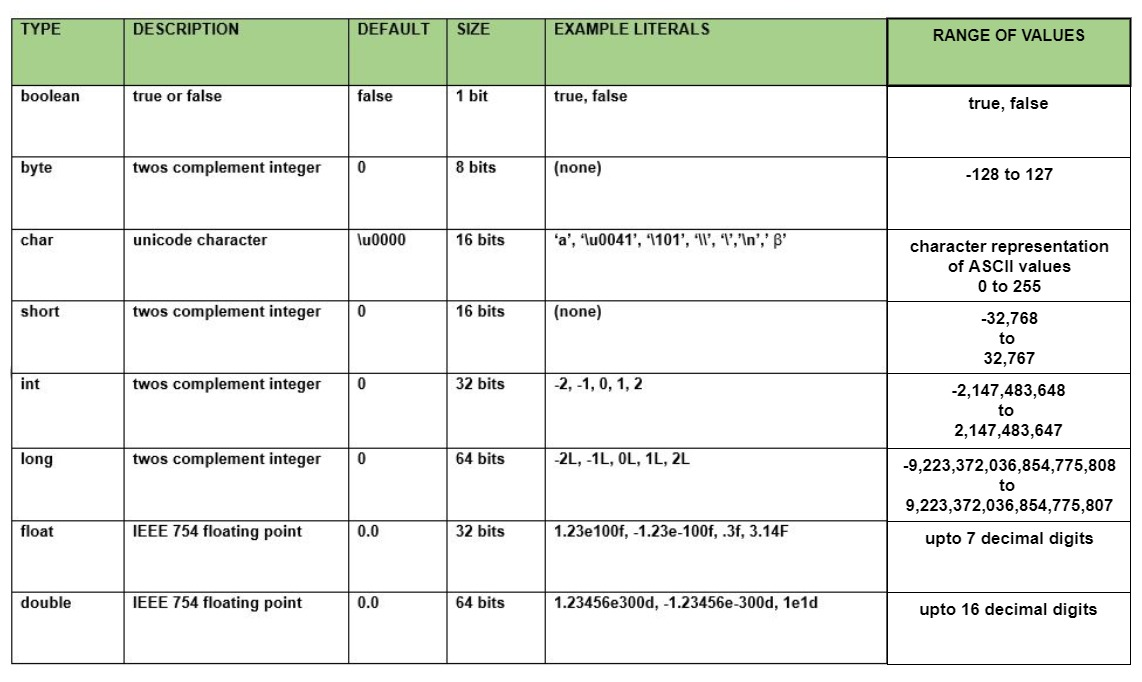Related Articles

# Data types in Java

• Difficulty Level : Easy
• Last Updated : 17 Feb, 2021

There are majorly two types of languages.

• First, one is Statically typed language where each variable and expression type is already known at compile time. Once a variable is declared to be of a certain data type, it cannot hold values of other data types.
Example: C, C++, Java.
• The other is Dynamically typed languages. These languages can receive different data types over time.
Example: Ruby, Python

Java is statically typed and also a strongly typed language because, in Java, each type of data (such as integer, character, hexadecimal, packed decimal, and so forth) is predefined as part of the programming language and all constants or variables defined for a given program must be described with one of the data types.
Data Types in JavaJava has two categories of data:

• Primitive Data Type: such as boolean, char, int, short, byte, long, float, and double
• Non-Primitive Data Type or Object Data type: such as String, Array, etc.

Primitive Data Type

Primitive data are only single values and have no special capabilities.There are 8 primitive data types

1. boolean: boolean data type represents only one bit of information either true or false, but the size of the boolean data type is virtual machine-dependent. Values of type boolean are not converted implicitly or explicitly (with casts) to any other type. But the programmer can easily write conversion code.

• Syntax:

```boolean booleanVar;
```
• Size:

```virtual machine dependent
```
• Values:

```true, false
```
• Default Value:

```false
```

## Java

 `// Java program to demonstrate boolean data type`` ` `class` `GeeksforGeeks {``    ``public` `static` `void` `main(String args[])``    ``{``        ``boolean` `b = ``true``;``        ``if` `(b == ``true``)``            ``System.out.println(``"Hi Geek"``);``    ``}``}`
1.
Output:

```Hi Geek

```

2. byte: The byte data type is an 8-bit signed two’s complement integer. The byte data type is useful for saving memory in large arrays.

• Syntax:

```byte byteVar;
```
• Size:

```1 byte ( 8 bits )
```
• Values:

```-128 to 127
```
• Default Value:
```0
```

## Java

 `// Java program to demonstrate byte data type in Java`` ` `class` `GeeksforGeeks {``    ``public` `static` `void` `main(String args[])``    ``{``        ``byte` `a = ``126``;`` ` `        ``// byte is 8 bit value``        ``System.out.println(a);`` ` `        ``a++;``        ``System.out.println(a);`` ` `        ``// It overflows here because``        ``// byte can hold values from -128 to 127``        ``a++;``        ``System.out.println(a);`` ` `        ``// Looping back within the range``        ``a++;``        ``System.out.println(a);``    ``}``}`
Output:
```126
127
-128
-127

```

3. short: The short data type is a 16-bit signed two’s complement integer. Similar to byte, use a short to save memory in large arrays, in situations where the memory savings actually matters.

• Syntax:

```short shortVar;
```
• Size:

```2 byte ( 16 bits )
```
• Values:

```-32, 768 to 32, 767 (inclusive)
```
• Default Value:

```0
```

4. int: It is a 32-bit signed two’s complement integer.

• Syntax:

```int intVar;
```
• Size:

```4 byte ( 32 bits )
```
• Values:

```-2, 147, 483, 648 to 2, 147, 483, 647 (inclusive)
```
• Default Value:

```0
```
• Note: In Java SE 8 and later, we can use the int data type to represent an unsigned 32-bit integer, which has value in the range [0, 232-1]. Use the Integer class to use int data type as an unsigned integer.

5. long: The long data type is a 64-bit two’s complement integer.

• Syntax:

```long longVar;
```
• Size:

```8 byte ( 64 bits )
```
• Values:
```-9, 223, 372, 036, 854, 775, 808
to
9, 223, 372, 036, 854, 775, 807
(inclusive)
```
• Default Value:

```0
```
• Note: In Java SE 8 and later, you can use the long data type to represent an unsigned 64-bit long, which has a minimum value of 0 and a maximum value of 264-1. The Long class also contains methods like comparing Unsigned, divide Unsigned, etc to support arithmetic operations for unsigned long.

6. float: The float data type is a single-precision 32-bit IEEE 754 floating-point. Use a float (instead of double) if you need to save memory in large arrays of floating-point numbers.

• Syntax:

```float floatVar;
```
• Size:

```4 byte ( 32 bits )
```
• Values:

```upto 7 decimal digits
```
• Default Value:

```0.0
```

7. double: The double data type is a double-precision 64-bit IEEE 754 floating-point. For decimal values, this data type is generally the default choice.

• Syntax:
```double doubleVar;
```
• Size:

```8 byte ( 64 bits )
```
• Values:

```upto 16 decimal digits
```
• Default Value:

```0.0
```
• Note: Both float and double data types were designed especially for scientific calculations, where approximation errors are acceptable. If accuracy is the most prior concern then, it is recommended not to use these data types and use BigDecimal class instead.
Please see this for details: Rounding off errors in Java

8. char: The char data type is a single 16-bit Unicode character.

• Syntax:

```char charVar;
```
• Size:

```2 byte ( 16 bits )
```
• Values:

```'\u0000' (0) to '\uffff' (65535)
```
• Default Value:

```'\u0000'
```

Why is the size of char is 2 byte in java..?
In other languages like C/C++ uses only ASCII characters and to represent all ASCII characters 8-bits is enough,
But java uses the Unicode system not the ASCII code system and to represent Unicode system 8 bit is not enough to represent all characters so java uses 2 bytes for characters.
Unicode defines a fully international character set that can represent most of the world’s written languages. It is a unification of dozens of character sets, such as Latin, Greeks, Cyrillic, Katakana, Arabic, and many more.

## Java

 `// Java program to demonstrate``// primitive data types in Java`` ` `class` `GeeksforGeeks {``    ``public` `static` `void` `main(String args[])``    ``{``        ``// declaring character``        ``char` `a = ``'G'``;`` ` `        ``// Integer data type is generally``        ``// used for numeric values``        ``int` `i = ``89``;`` ` `        ``// use byte and short``        ``// if memory is a constraint``        ``byte` `b = ``4``;`` ` `        ``// this will give error as number is``        ``// larger than byte range``        ``// byte b1 = 7888888955;`` ` `        ``short` `s = ``56``;`` ` `        ``// this will give error as number is``        ``// larger than short range``        ``// short s1 = 87878787878;`` ` `        ``// by default fraction value``        ``// is double in java``        ``double` `d = ``4.355453532``;`` ` `        ``// for float use 'f' as suffix``        ``float` `f = ``4``.7333434f;`` ` `        ``System.out.println(``"char: "` `+ a);``        ``System.out.println(``"integer: "` `+ i);``        ``System.out.println(``"byte: "` `+ b);``        ``System.out.println(``"short: "` `+ s);``        ``System.out.println(``"float: "` `+ f);``        ``System.out.println(``"double: "` `+ d);``    ``}``}`
Output:
```char: G
integer: 89
byte: 4
short: 56
float: 4.7333436
double: 4.355453532

```

Non-Primitive Data Type or Reference Data Types

The Reference Data Types will contain a memory address of variable value because the reference types won’t store the variable value directly in memory. They are strings, objects, arrays, etc.

• String: Strings are defined as an array of characters. The difference between a character array and a string in Java is, the string is designed to hold a sequence of characters in a single variable whereas, a character array is a collection of separate char type entities.
• Unlike C/C++, Java strings are not terminated with a null character.
Below is the basic syntax for declaring a string in Java programming language.
Syntax:

```<String_Type> <string_variable> = “<sequence_of_string>”;

```
• Example:

```// Declare String without using new operator
String s = "GeeksforGeeks";

// Declare String using new operator
String s1 = new String("GeeksforGeeks");

```
• Class: A class is a user-defined blueprint or prototype from which objects are created.  It represents the set of properties or methods that are common to all objects of one type. In general, class declarations can include these components, in order:
1. Modifiers: A class can be public or has default access (Refer this for details).
2. Class name: The name should begin with a initial letter (capitalized by convention).
3. Superclass(if any): The name of the class’s parent (superclass), if any, preceded by the keyword extends. A class can only extend (subclass) one parent.
4. Interfaces(if any): A comma-separated list of interfaces implemented by the class, if any, preceded by the keyword implements. A class can implement more than one interface.
5. Body: The class body surrounded by braces, { }.
• Object: It is a basic unit of Object-Oriented Programming and represents the real-life entities.  A typical Java program creates many objects, which as you know, interact by invoking methods. An object consists of :
1. State: It is represented by attributes of an object. It also reflects the properties of an object.
2. Behavior: It is represented by methods of an object. It also reflects the response of an object with other objects.
3. Identity: It gives a unique name to an object and enables one object to interact with other objects.
• Interface: Like a class, an interface can have methods and variables, but the methods declared in an interface are by default abstract (only method signature, nobody).
• Interfaces specify what a class must do and not how. It is the blueprint of the class.
• An Interface is about capabilities like a Player may be an interface and any class implementing Player must be able to (or must implement) move(). So it specifies a set of methods that the class has to implement.
• If a class implements an interface and does not provide method bodies for all functions specified in the interface, then the class must be declared abstract.
• A Java library example is, Comparator Interface. If a class implements this interface, then it can be used to sort a collection.
• Array: An array is a group of like-typed variables that are referred to by a common name. Arrays in Java work differently than they do in C/C++. The following are some important points about Java arrays.
• In Java, all arrays are dynamically allocated. (discussed below)

• Since arrays are objects in Java, we can find their length using member length. This is different from C/C++ where we find length using size.
• A Java array variable can also be declared like other variables with [] after the data type.
• The variables in the array are ordered and each has an index beginning from 0.
• Java array can be also be used as a static field, a local variable or a method parameter.
• The size of an array must be specified by an int value and not long or short.
• The direct superclass of an array type is Object

• Every array type implements the interfaces Cloneable and java.io.Serializable.

Quiz on Data Type in Java
This article is contributed by Shubham Agrawal. If you like GeeksforGeeks and would like to contribute, you can also write an article using contribute.geeksforgeeks.org or mail your article to contribute@geeksforgeeks.org. See your article appearing on the GeeksforGeeks main page and help other Geeks.